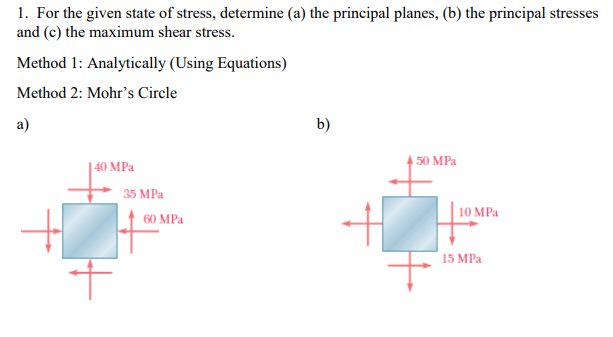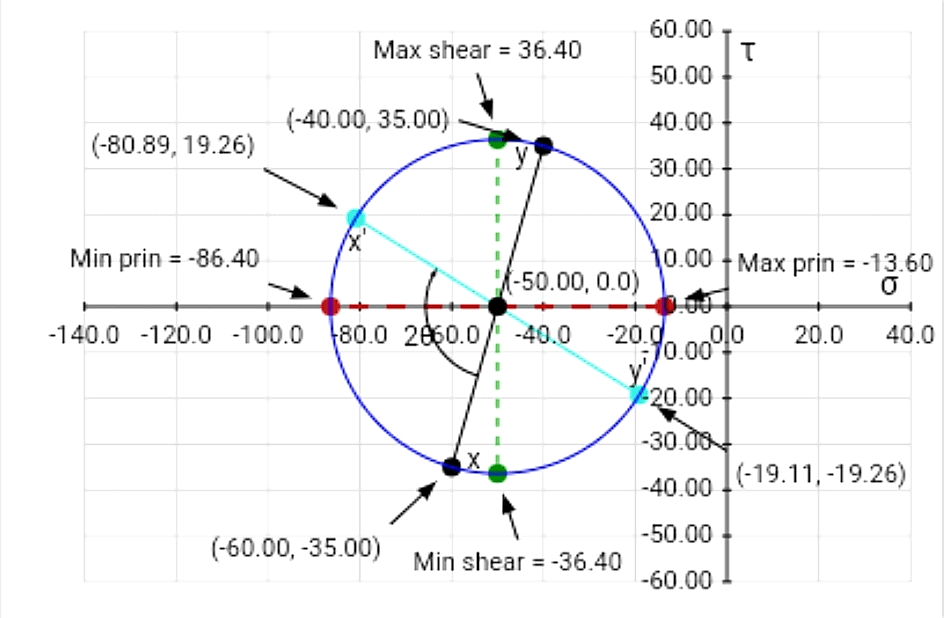# 1. For the given state of stress, determine (a) the principal planes, (b) the principal stresses and (c) the maximum shear st 1. For the given state of stress, determine (a) the principal planes, (b) the principal stresses and (c) the maximum shear stress. Method 1: Analytically (Using Equations) Method 2: Mohr's Circle

Question-AnswerCategory: Strength of Materials1. For the given state of stress, determine (a) the principal planes, (b) the principal stresses and (c) the maximum shear st 1. For the given state of stress, determine (a) the principal planes, (b) the principal stresses and (c) the maximum shear stress. Method 1: Analytically (Using Equations) Method 2: Mohr's Circle1. For the given state of stress, determine (a) the principal planes, (b) the principal stresses and (c) the maximum shear stress. Method 1: Analytically (Using Equations) Method 2: Mohr’s Circle